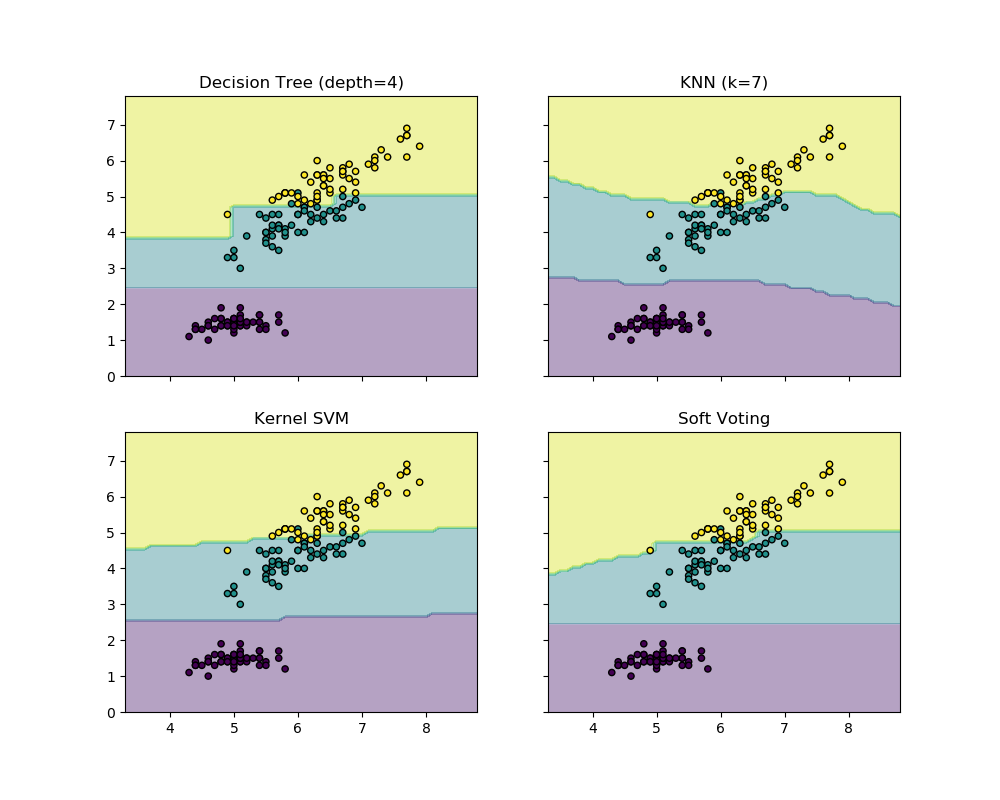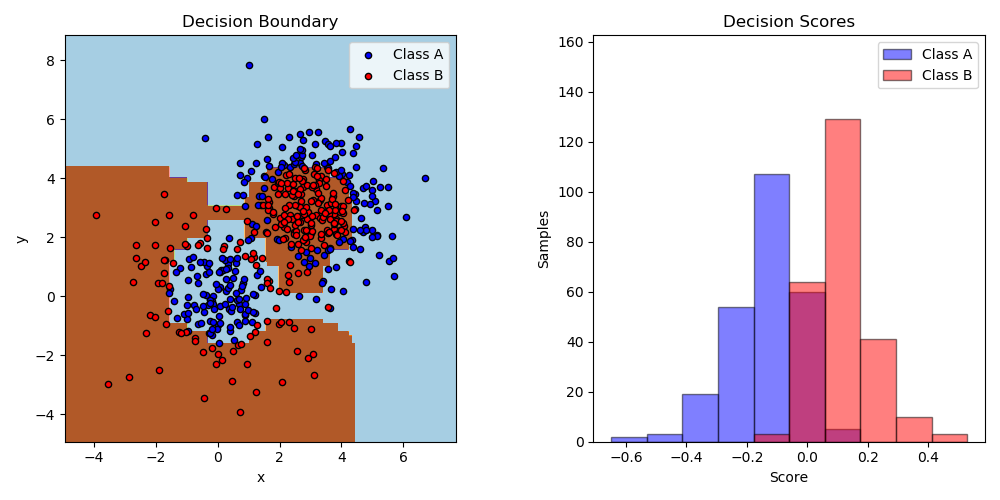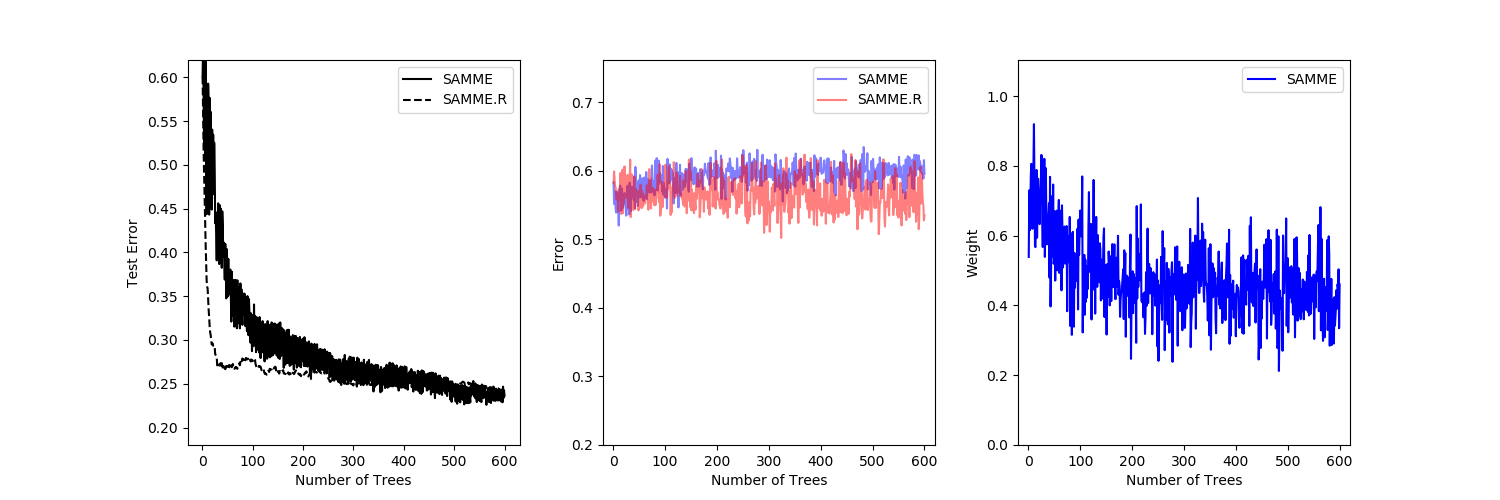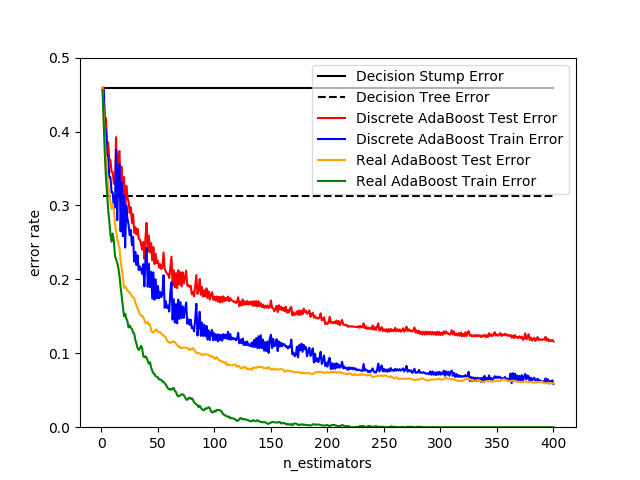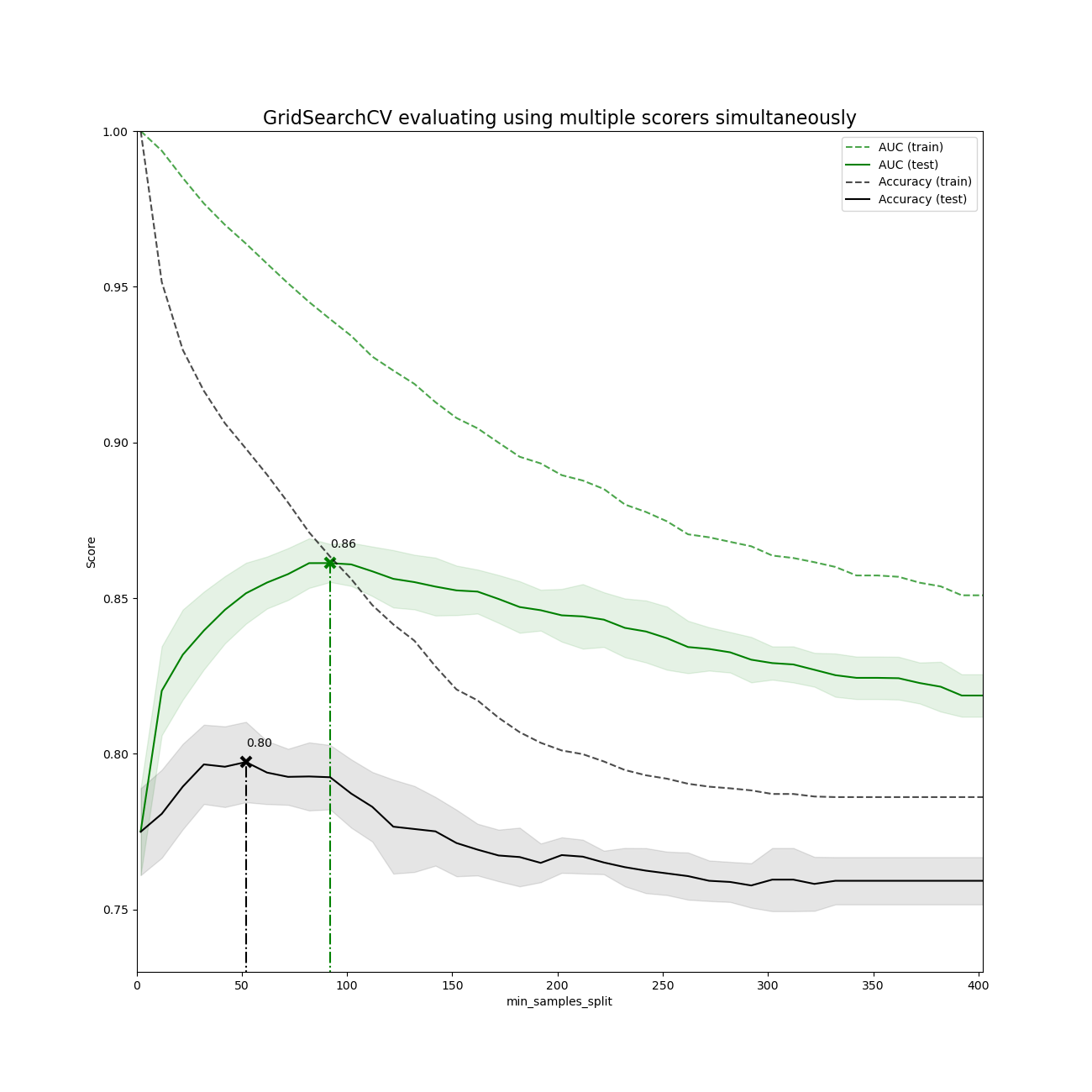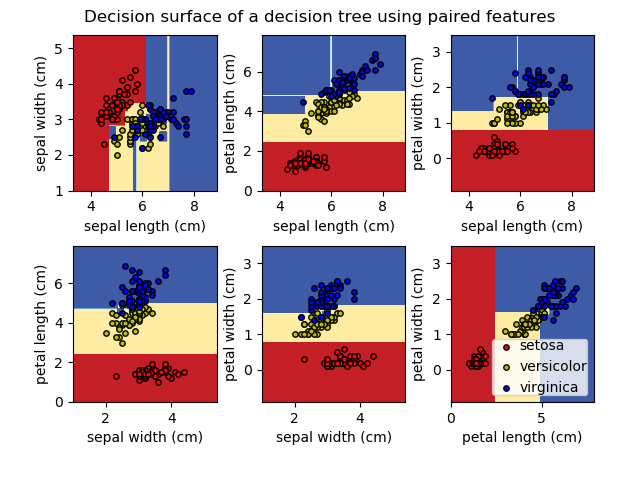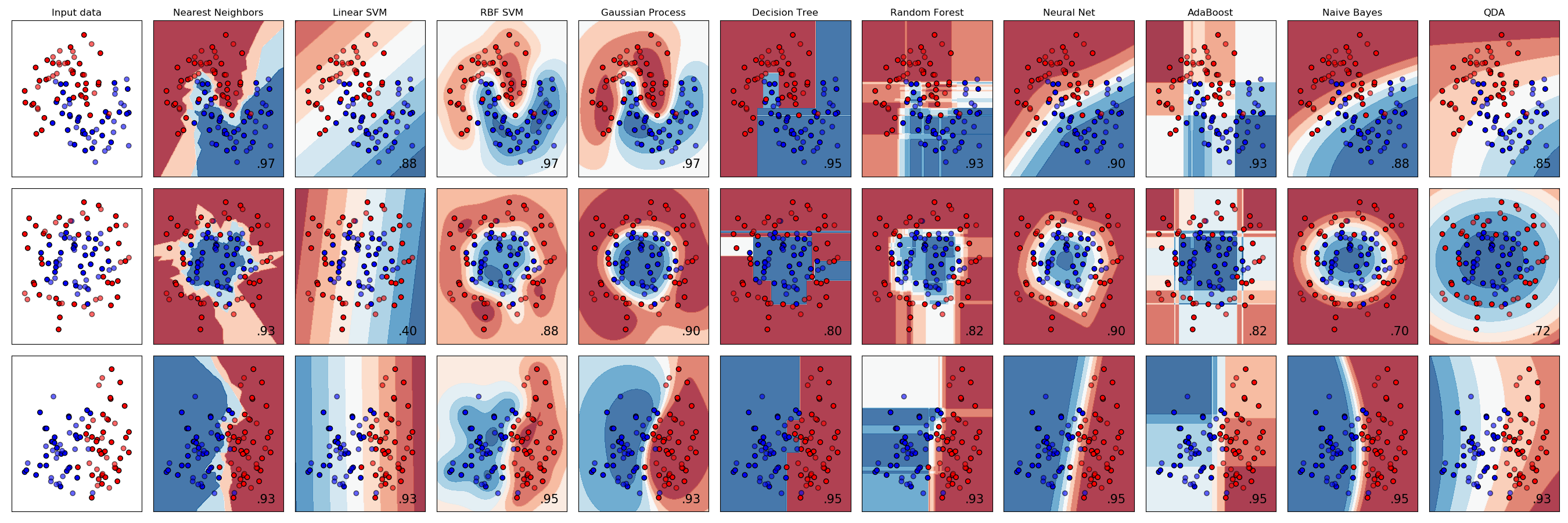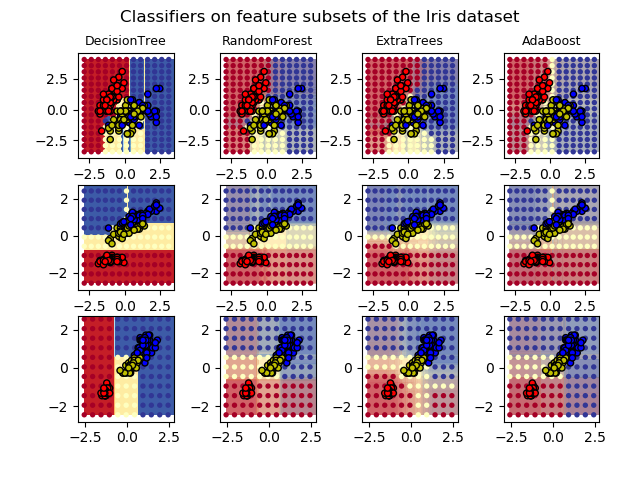# sklearn.tree.DecisionTreeClassifier¶

class sklearn.tree.DecisionTreeClassifier(*, criterion='gini', splitter='best', max_depth=None, min_samples_split=2, min_samples_leaf=1, min_weight_fraction_leaf=0.0, max_features=None, random_state=None, max_leaf_nodes=None, min_impurity_decrease=0.0, min_impurity_split=None, class_weight=None, presort='deprecated', ccp_alpha=0.0)

[源码]

criterion {“gini”, “entropy”}, default=”gini”

splitter {“best”, “random”}, default=”best”

max_depth int, default=None

min_samples_split int or float, default=2

· 如果取值 int , 则将min_samples_split视为最小值。
· 如果为float，则min_samples_split是一个分数，而ceil（min_samples_split * n_samples）是每个拆分的最小样本数。

-注释 在版本0.18中更改：增加了分数形式的浮点值。
min_samples_leaf int or float, default=1

· 如果为int，则将min_samples_leaf视为最小值
· 如果为float，则min_samples_leaf是一个分数，而ceil（min_samples_leaf * n_samples）是每个节点的最小样本数。

- 注释： 在版本0.18中发生了更改：添加了分数形式的浮点值。
min_weight_fraction_leaf float, default=0.0

max_features int, float or {“auto”, “sqrt”, “log2”}, default=None

- 如果为int，则在每次拆分时考虑max_features功能。
- 如果为float，则max_features是一个分数，而int（max_features * n_features）是每个分割处的特征数量。
- 如果为“auto”，则max_features = sqrt（n_features）
- 如果为“sqrt”，则max_features = sqrt（n_features）
- 如果为“log2”，则max_features = log2（n_features）
- 如果为None，则max_features = n_features

random_state int, RandomState instance, default=None

max_leaf_nodes int, default=None

min_impurity_decrease float, default=0.0

N_t / N * (impurity - N_t_R / N_t * right_impurity
- N_t_L / N_t * left_impurity)

min_impurity_split float, default=0

class_weight dict, list of dict or “balanced”, default=None
{class_label: weight}的形式表示与类别关联的权重。如果取值None,所有分类的权重为1。对于多输出问题，可以按照y的列的顺序提供一个字典列表。

“平衡”模式使用y的值自动将权重与输入数据中的类频率成反比地调整为n_samples /（n_classes * np.bincount（y））

presort deprecated, default=’deprecated’

ccp_alpha non-negative float, default=0.0

classes_ ndarray of shape (n_classes,) or list of ndarray

feature_importances_ ndarray of shape (n_features,)

max_features_ int
max_features 的推断值。
n_classes_ int or list of int

n_features_ int

n_outputs_ int

tree_ Tree

DecisionTreeRegressor 一个回归决策树.

1、https://en.wikipedia.org/wiki/Decision_tree_learning

2、L. Breiman, J. Friedman, R. Olshen, and C. Stone, “Classification and Regression Trees”, Wadsworth, Belmont, CA, 1984.

3、T. Hastie, R. Tibshirani and J. Friedman. “Elements of Statistical Learning”, Springer, 2009.

4、L. Breiman, and A. Cutler, “Random Forests”, https://www.stat.berkeley.edu/~breiman/RandomForests/cc_home.htm

>>> from sklearn.datasets import load_iris>>> from sklearn.model_selection import cross_val_score>>> from sklearn.tree import DecisionTreeClassifier>>> clf = DecisionTreeClassifier(random_state=0)>>> iris = load_iris()>>> cross_val_score(clf, iris.data, iris.target, cv=10)...                             # doctest: +SKIP...array([ 1.     ,  0.93...,  0.86...,  0.93...,  0.93...,        0.93...,  0.93...,  1.     ,  0.93...,  1.      ])

apply(X[, check_input]) 返回每个叶子节点上被预测样本的索引。
cost_complexity_pruning_path(X, y[, …]) 在最小化成本复杂性修剪期间计算修剪路径 。
decision_path(X[, check_input]) 返回决策树的决策路径。
fit(X, y[, sample_weight, check_input, …]) 根据训练集（X，y）建立决策树分类器。
get_depth() 返回决策树的深度 。
get_n_leaves() 返回决策树的叶子数。
get_params([deep]) 获取此估算器的参数。
predict(X[, check_input]) 预测X的类别或回归值。
predict_log_proba(X) 预测输入样本X的类对数概率。
predict_proba(X[, check_input]) 预测输入样本X的类别概率。
score(X, y[, sample_weight]) 返回给定测试数据和标签上的平均准确度。
set_params(**params) 设置此估算器的参数。
__init__(*, criterion='gini', splitter='best', max_depth=None, min_samples_split=2, min_samples_leaf=1, min_weight_fraction_leaf=0.0, max_features=None, random_state=None, max_leaf_nodes=None, min_impurity_decrease=0.0, min_impurity_split=None, class_weight=None, presort='deprecated', ccp_alpha=0.0)

[源码]

apply(X, check_input=True)

[源码]

X {array-like, sparse matrix} of shape (n_samples, n_features)

check_input bool, default=True

X_leaves array-like of shape (n_samples,)

cost_complexity_pruning_path(X, y, sample_weight=None)

[源码]

X {array-like, sparse matrix} of shape (n_samples, n_features)

y array-like of shape (n_samples,) or (n_samples, n_outputs)

sample_weight array-like of shape (n_samples,), default=None

ccp_path Bunch

ccp_alphas ndarray

impurities ndarray

decision_path(X, check_input=True)

[源码]

X {array-like, sparse matrix} of shape (n_samples, n_features)

check_input bool, default=True

indicator sparse matrix of shape (n_samples, n_nodes)

property feature_importances_

feature_importances_ ndarray of shape (n_features,)

fit(X, y, sample_weight=None, check_input=True, X_idx_sorted=None)

[源码]

X {array-like, sparse matrix} of shape (n_samples, n_features)

y array-like of shape (n_samples,) or (n_samples, n_outputs)

sample_weight array-like of shape (n_samples,), default=None

check_input bool, default=True

X_idx_sorted array-like of shape (n_samples, n_features), default=None

self DecisionTreeClassifier

get_depth()

[源码]

self.tree_.max_depth int

get_n_leaves()

[源码]

self.tree_.n_leaves int

get_params(deep=True)

[源码]

deep bool, default=True

params mapping of string to any

predict(X, check_input=True)

[源码]

X {array-like, sparse matrix} of shape (n_samples, n_features)

check_input bool, default=True

y array-like of shape (n_samples,) or (n_samples, n_outputs)

predict_log_proba(X)

[源码]

X {array-like, sparse matrix} of shape (n_samples, n_features)

proba ndarray of shape (n_samples, n_classes) or list of n_outputs such arrays if n_outputs > 1

predict_proba(X, check_input=True)

[源码]

X {array-like, sparse matrix} of shape (n_samples, n_features)

check_input bool, default=True

proba ndarray of shape (n_samples, n_classes) or list of n_outputs such arrays if n_outputs > 1

score(X, y, sample_weight=None)

[源码]

X array-like of shape (n_samples, n_features)

y array-like of shape (n_samples,) or (n_samples, n_outputs)
X的真实标签
sample_weight array-like of shape (n_samples,), default=None

score float
self.predict(X) wrt. y的平均准确度
set_params(**params)

[源码]

**params dict

self object

## sklearn.tree.DecisionTreeClassifier使用示例¶